International Journal on Data Science and Technology
Volume 2, Issue 1, January 2016, Pages: 15-20

Efficient Computation of Binomial Coefficients Using Splay Trees

Vinayshekhar Bannihatti Kumar, Karthik Radhakrishnan, Aman Kishore Achpal

Computer Science Department, PES Institute of Technology, Bangalore, India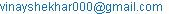(V. B. Kumar)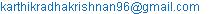(K. Radhakrishnan)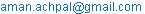(A. K. Achpal)

Vinayshekhar Bannihatti Kumar, Karthik Radhakrishnan, Aman Kishore Achpal. Efficient Computation of Binomial Coefficients Using Splay Trees. International Journal on Data Science and Technology. Vol. 2, No. 1, 2016, pp. 15-20. doi: 10.11648/j.ijdst.20160201.14

Abstract: Combinatorics is an important branch of mathematics. Binomial coefficients play an important role in the computation of permutations and combinations in mathematics. This paper describes a novel method of computing coefficients using Splay Trees. The performance in terms of space as well as time efficiency is compared, and conclusions on the technique are offered. We show how this technique is particularly effective in the expansion of a binomial expression.

Keywords: Binomial Coefficients, Splay Trees, Pascal’s Triangle, Memoization, Dynamic Programming, Combinatorics

Contents

1. Introduction

Binomial coefficients have many specific and generic applications in mathematics as well as in computation, including computation of Catalan numbers and in statistics . Additionally, binomial coefficients are used in multiplication of large numbers using binomial expansion, and are used in generating all possible permutations/combinations of sets. Therefore, owing to the cascading benefits, it is important to find an efficient method of computing binomial coefficients. The array implementation of binomial coefficients is a classic dynamic programming technique. In this paper, we explore a novel method of using a Splay Tree to compute binomial coefficients, as opposed to using an array. Furthermore, we discuss memory and space optimizations that have been implemented to further enhance the performance of the Splay Tree. Finally, we perform a comparative analysis of the performance of our technique alongside conventional arrays and the Binary Search Tree.

The remainder of the paper is structured in the following manner: Section II describes the past work done in the field, Section III discusses the mathematical and computational foundations underlying our method, Section IV describes our framework as well as our algorithm, Section V contains an analysis of the results in comparison to other techniques, section VI describes how this technique specifically excels in the expansion of binomial expressions, and finally Section VII offers conclusions and direction for future work.

2. Past Work

Splay Tree is a data structure which is ideal for caching. Because of its insert and search rules Splay Trees have been used for this purpose. Eric K. Lee and Charles U. Martel have described in their paper  some applications splay trees are most suited for, such as faster query execution using better cache management. Subrata Mondal used Splay Trees in Cache replacement Algorithms . Splay Trees have been found in the field of networking as well. Dynamic Scheme for Packet Classification was done by Nizar Ben Neji and Adel Bouhoula . Wei Zhou, Zilong Tan, Shaowen Yao, and Shipu Wang proposed their work on Efficient Resource Location in P2P Networks . Splay Trees have also been used in Data Compression Algorithms. D. W Jones in his work proposes the use of splay trees in arithmetic data compression . Splay Trees were used in order to make Distribution Systems Evaluation simpler . Splay Trees were used as cumulative frequency tables in order to maintain Dynamic arithmetic data compression . Splay Trees were also used in memory hash tables for accumulating text vocabularies . In a new approach proposed by us we use splay trees in a branch of mathematics for computing binomial coefficients.

3. Mathematical and Computational Foundations

A.   Splay Trees

Splay trees are self – adjusting binary search trees , i. e. they have special insert and search rules. When a node is being accessed for retrieval or insertion or deletion, special rotations  are performed on the tree, resulting in the newly accessed node becoming the root of the modified tree. This technique is called splaying and the tree derives its name from this operation. These rotations will ensure that nodes that are frequently accessed will always be closer to the root whereas unused nodes will get splayed away from the root. When a node is accessed, either a single rotation or a series of rotations are applied to move the node towards the root. The advantage of using Splay trees is that it does not require height or balance factors as in other trees (e. g. AVL trees, Red-Black trees.) Informally, one can think of the splay trees as implementing a sort of ‘most recently used’ policy on tree accesses. The nodes used most recently will come closer to the root and the ones not used recently will be away from the root. This is done because the tree dynamically adjusts itself after every insertion or search. This makes splay trees ideal for caching. Recently used nodes are closer to the root making splay tree lookup quicker than normal binary search tree lookup for these nodes.

B.  Pascal’s Triangle

In mathematics, Pascal's triangle is a triangular array of the binomial coefficients . It is named after French mathematician Blaise Pascal, Other mathematicians have studied similar pattern centuries before him in India (Bhaskaracharya, Pingala etc.), Iran, China, Germany, and Italy.

The rows of Pascal's triangle are conventionally enumerated starting with row n = 0 at the top (the 0th row). The entries in each row are numbered from the left beginning with k = 0 and are usually staggered relative to the numbers in the adjacent rows. Having the indices of both rows and columns start at zero makes it possible to state that the binomial coefficient appears in the nth row and kth column of Pascal's triangle. A simple construction of the triangle proceeds in the following manner: In row 0, the topmost row, the entry is (the entry is in the zeroth row and zeroth column). Then, to construct the elements of the following rows, add the number above and to the left with the number above and to the right of a given position to find the new value to place in that position. If either the number to the right or left is not present, substitute a zero in its place. For example, the initial number in the first (or any other) row is 1 (the sum of 0 and 1), whereas the numbers 1 and 3 in the third row are added to produce the number 4 in the fourth row.

4. Framework and Algorithm

C.  System Architecture

Figure 1 describes the architecture of our system in the form of a flow chart. Each stage is subsequently explained in further detail, along with the optimizations that have been implemented to further enhance the performance.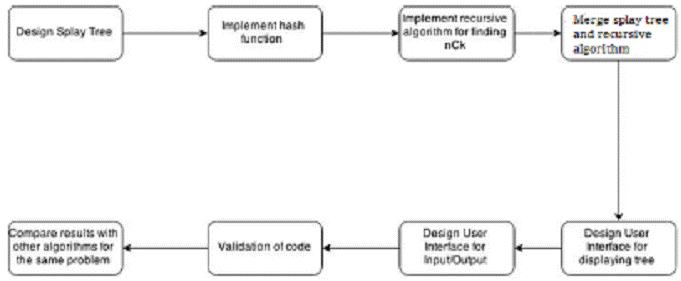Figure 1. System Architecture.

D.  Design of Splay Tree

Splay Tree Node (STN): The Splay tree comprises of nodes with links between them. Each such splay tree node has fields’ n and k, A BigInteger object storing the value of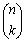(A BigInteger object is needed as the values ofbecome very large when the values of n and k are large). E. g. Value of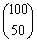spans 300 digits.) An element which is the unique key of a particular node is obtained from a hashing function described below. Every node also includes links to its left and right children and a link to its parent node.

Splay Tree (ST): The Splay Tree comprises of several STNs which are connected to the root node which is initially set to null. ST contains the insert, search and splaying methods. The insertion does a BST type insertion first and then calls the splay method. The splay method performs one or more rotations, to either make left child parent or right child parent, to move the last inserted node towards the root. Search or lookup method performs a binary search and splays the last non-null node encountered in the search, all the way to the root. Thus, the most recently used nodes are always closer to the root.

It contains the hashing function and array of prime numbers used by the hashing function, counter for number of nodes in a tree.

E.  Hash Function

We use a hash technique to map every uniquepair to a single unique number. This number serves as the key to a STN for insertion and search operations. This is achieved by mapping natural numbers to prime numbers. The nth natural number is mapped to the nth prime number. For example 7 is mapped to the 7th prime number which is 19. Hence we can map every value of n and k to a pair of prime numbers and for every unordered pair n and k, the product of these prime numbers is always unique and hence serves as a key for the splay tree. This hash function also ensures that there is no collision.

F.   Recursive algorithm to findThe third step is to implement the algorithm for findingrecursively and merge it with the splay tree as the lookup table. The algorithm implemented is:

binomial(int n, int k) {

if (k > n/2)

k = n - k;

if (k == 0)

return 1;

if (k == 1)

return n; }

v = table. search (n, k); // lookup cache table

if (v != null) // if value was previously cached

return v. result(); // return cached value

v = binomial(n-1, k-1) + binomial (n-1, k); // compute

table. insert(n, k, v); // and cache the result

return v;

The recursive formula underlying the algorithm is as follows:=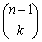+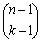G.  Memory Optimizations

To optimize memory, only the splay nodes used in the computation of a particular n and k, (instead of all cells within n*k in array implementation), are stored.=+The formula has n & k defined in terms of previous values, n-1 and k-1. To avoid re-computation, the previous results can be cached in a global table in a recursive implementation. This technique called memoization has the further advantage of speeding up future invocations as long as the table (declared global) persists between calls. The table is implemented as a splay tree in our implementation.

Generally calculating large Binomial Co-efficient takes significant time and memory, but with memoization technique and implementing using Splay Trees, the Binomial Coefficients are calculated in more efficient manner.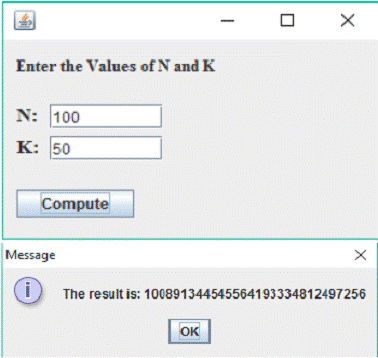Figure 2. User Interface.

H. User Interface

The user interface is implemented using Java’s SWING libraries. A window pops up containing input fields for N and K. A button to calculate the value ofis provided. The result is displayed with the help of JOptionPane. Such large numbers were represented with the help of Big Integer Class of Java. Additionally, we have also created an applet so as to be able to access the project from a website also. The user interface is shown in Figure 2.

5. Analysis of Results

The testing was carried out on a machine powered by Intel Core i3 processor with a clock speed of 2.2GHz and 4 gigabytes of RAM. We compare the time and space efficiency of our method to existing techniques.

I.    Space Efficiency

The splay tree method is more space efficient. In the 2-dimensional array of Pascal’s triangle, memory is allocated for all cells even if they aren’t needed. However in ST implementation, only the necessary cell values are stored as splay tree nodes (STN). For example, to calculate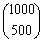, the array implementation requires 1000*500=5X105 nodes while the splay tree implementation only uses 124750 nodes being lot more space efficient (75% more efficient). This is a big advantage while calculating extremely large coefficients. E. g.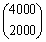can be computed by the splay tree implementation method on a 4GB machine whereas the array implementation runs out of memory.

J.    Time Analysis

The branch of algorithmics is primarily concerned with time. Various known methods were implemented and compared, the results were computed, and time taken versus input size was plotted.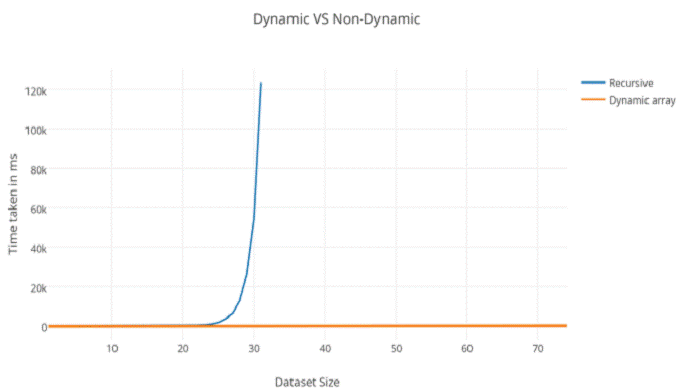Figure 3. Impact of Memoization.

6. Impact of Memoization

The first metric that needs to be assessed is if whether memoization has had any impact in improving the efficiency of the system. Figure 3 compares the system with and without memoization.

It is observed that the system runs significantly faster when memoization is used. The reasons for the same can be explained using Figure 4, where we show how repeated calculations are avoided, when memoization is used.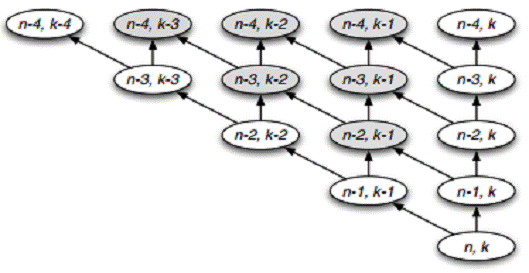Figure 4. Repeated Computation in Binomial Coefficient calculation.

Without memoization, the shaded nodes are recomputed multiple times making computation expensive, and the execution extremely slow. Figure 3 embodies these results: - The blue line (recursive implementation without memoization) has a near vertical rise, and proves that without memoization, calculation of binomial coefficients is impossible for even moderately large values. In comparison, the orange line (Dynamic array implementation using memoization) shows feasibility in computing even very large values.

7. Comparison with Conventional Array Implementation

In order to compare the conventional technique against ours,was computed for all valid values of k, with n varying from n=1 to n=200. The time plotted for each calculation was tabulated, and the results of the tabulation are plotted in Figure 5. It can be inferred from the graph that our method performs faster than the array implementation, and is more time efficient. Therefore, our method outperforms the conventional implementation in both time and space efficiency. The obtained results have been plotted in Figure 5, where time of computation is compared against dataset size.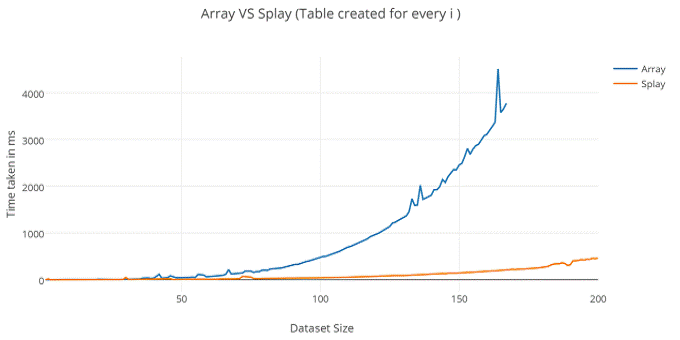Figure 5. Array Implementation Versus Splay Tree Implementation.

8. Comparison with Binary Search Tree

We compared the performance of splay trees against a Binary search tree using the same methodology, and obtained the graph plotted in Figure 6. We see that splay trees are clearly faster, and the reasons for the same are as follows: The nature of the problem matches the structure of a splay tree, as splaying causes the most recently used nodes to come to the top, thereby making access faster. Splay trees are also easier to implement and faster than other such trees such as red black which needs colouring of nodes or AVL trees which requires computing balance factors .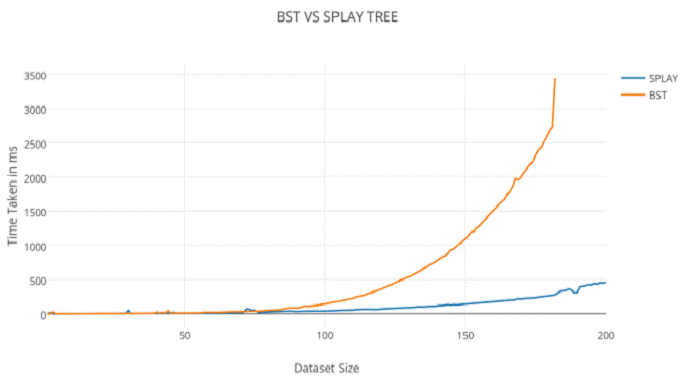Figure 6. Binary Search Tree Implementation Versus Splay Tree Implementation.

9. Application

Our technique is particularly useful when the intermediate results are important as well. One such scenario in which this occurs is binomial expansion . Regardless of the expansion, only a single splay tree is created.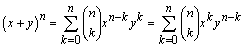By creating the tree only once, all the binomial coefficients in the binomial expansion can be obtained much quicker than the Array implementation of Pascal’s triangle, and hence, the splay tree outperforms the array implementation and can be used to cache intermediate results of a binomial expansion. It is also noteworthy that the creation of the splay tree helps when next coefficient in line is to be calculated.

10. Conclusion and Future Work

In this paper, we have explored computation of Binomial Coefficients using Splay Trees to cache the intermediate results. This implementation is efficient both in memory and running time. The runtime performance is favorable compared to Pascal’s triangle, in which results are stored in an array. Furthermore, the Splay Trees requires less space by storing only those nodes that are required. We have also compared the performance with other implementations including the binary search tree. From these comparisons, we have shown that using splay trees, Binomial Expansions can be computed efficiently. The tabulation of our results can be found in Figure 7.

Future work in the field includes further improving performance by using a Randomized Splay Tree as opposed to the conventional implementation of the splay tree, as proposed by Susanne Albers and Marek Karpinski .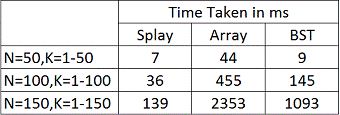Figure 7. Comparison Between Various Implementations.

Acknowledgement

We would like to thank Professor Srinivasa Murthy from Computer Science Department of P. E. S Institute of technology for his continued guidance and support, without whom this project would have been difficult to complete.

References

1. Wikipedia, "Binomial Theorem", 2015. [Online]. Available: https://en.wikipedia.org/wiki/Binomial_theorem. [Accessed: 19- Dec- 2015].
2. E. Lee and C. Martel, "When to use splay trees", Softw: Pract. Exper., vol. 37, no. 15, pp. 1559-1575, 2007.
3. Mondal, Subrata. (2013). Study of Splay Tree for use in Cache Replacement Algorithm. (Master’s thesis, Jadavpur University, West Bengal, India.)
4. N. Neji and A. Bouhoula, "Dynamic Scheme for Packet Classification", Advances in Soft Computing, vol. 53, pp. 211-218, 2009.
5. W. Zhou, Z. Tan, S. Yao and S. Wang, "A Splay Tree-Based Approach for Efficient Resource Location in P2P Networks", the Scientific World Journal, vol. 2014, pp. 1-11, 2014.
6. D. Jones, "Application of splay trees to data compression", Communications of the ACM, vol. 31, no. 8, pp. 996-1007, 1988.
7. É. Rivièr and P. Felber, "SPLAY: Distributed Systems Evaluation Made Simple", USENIX symposium on Networked systems design and implementation, vol. 6, pp. 185-198, 2009.
8. P. Fenwick, "A new data structure for cumulative frequency tables", Softw: Pract. Exper., vol. 24, no. 3, pp. 327-336, 1994.
9. J. Zobel, S. Heinz and H. Williams, "In-memory hash tables for accumulating text vocabularies", Information Processing Letters, vol. 80, no. 6, pp. 271-277, 2001.
10. Wikipedia, "Splay tree", 2015. [Online]. Available: https://en.wikipedia.org/wiki/Splay_tree. [Accessed: 19- Dec- 2015].
11. Mount, David. "Splay Trees", University of Maryland, 2001. Lecture.
12. J. Grossman, K. Rosen and J. Grossman, Student's solutions guide to accompany discrete mathematics and its applications. New York: McGraw-Hill, 2012.
13. B. Pfaff, "Performance analysis of BSTs in system software", ACM SIGMETRICS Performance Evaluation Review, vol. 32, no. 1, p. 410, 2004.
14. S. Albers, "Randomized splay trees: Theoretical and experimental results", Information Processing Letters, vol. 81, no. 4, pp. 213-221, 2002.

 Contents 1. 2. 3. 4. 5. 6. 7. 8. 9. 10.
Article ToolsAbstractPDF(1443K)